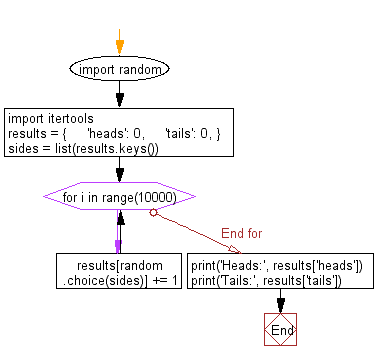﻿ Python Math: Flip a coin 1000 times and count heads and tails - w3resource# Python Math: Flip a coin 1000 times and count heads and tails

## Python Math: Exercise-53 with Solution

Write a Python program to flip a coin 1000 times and count heads and tails.

Sample Solution:-

Python Code:

``````import random
import itertools

results = {
'tails': 0,
}
sides = list(results.keys())

for i in range(10000):
results[random.choice(sides)] += 1

print('Tails:', results['tails'])
```
```

Sample Output:

```Heads: 5037
Tails: 4963
```

Pictorial Presentation:Flowchart:## Visualize Python code execution:

The following tool visualize what the computer is doing step-by-step as it executes the said program:

Python Code Editor:

Have another way to solve this solution? Contribute your code (and comments) through Disqus.

What is the difficulty level of this exercise?

Test your Programming skills with w3resource's quiz.

﻿

```>>> students = [{'name': 'John', 'score': 98}, {'name': 'Mike', 'score': 94}, {'name': 'Jennifer', 'score': 99}]